Courses

# RS Latch: S-R Flip Flops Notes | EduRev

## Electrical Engineering (EE): RS Latch: S-R Flip Flops Notes | EduRev

The document RS Latch: S-R Flip Flops Notes | EduRev is a part of the Electrical Engineering (EE) Course Digital Electronics.
All you need of Electrical Engineering (EE) at this link: Electrical Engineering (EE)

## The RS Latch

Flip-flops can also be considered as latch circuits due to them remembering or ‘latching’ a change at their inputs. A common form of RS latch is shown in Fig. 5.2.5. In this circuit the S and R inputs have now become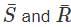inputs, meaning that they will now be ‘active high’.

They have also changed places, the R input is now on the gate having the Q output and the S input is on the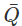gate. These changes occur because the circuit is using NOR gates instead of NAND.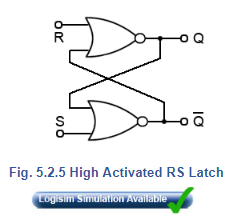## RS Latch Truth Table (Table 5.2.2)

1. Q is set to 1 when the S input goes to logic 1.
2. This is remembered on Q after the S input returns to logic 0.
3. Q is reset set to 0 when the R input goes to logic 1.
4. This is remembered on Q after the R input returns to logic 0.
5. If both inputs are at logic 1, Q is the same as Q (the non-allowed state).
6. The state of the outputs cannot be guaranteed if the inputs change from 1,1 to 0, 0 at the same time.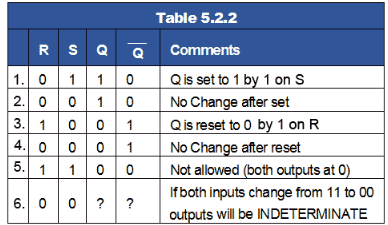## Timing Diagrams

Truth tables are not always the best method for describing the action of a sequential circuit such as the SR flip-flop. Timing diagrams, which show how the logic states at various points in a circuit vary with time, are often preferred.

Fig. 5.2.6 shows a timing diagram describing the action of the basic RS Latch for logic changes at R and S. At time (a) S goes high and sets Q, which remains high until time (b) when S is low and R goes high, resetting Q. During period (c) both S and R are high causing the non-allowed state where both outputs are high. After period (c) Q remains high until time (d) when R goes high, resetting Q. Period (e) is another non-allowed period, at the end of which both inputs go low causing an indeterminate output condition in period (f).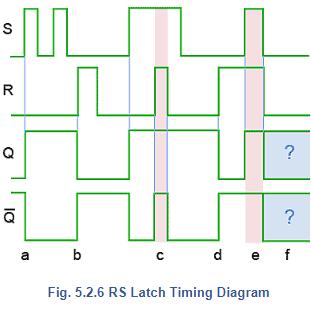## The Clocked SR Flip-flop

Fig. 5.2.7 shows a useful variation on the basic SR flip-flop, the clocked SR flip-flop. By adding two extra NAND gates, the timing of the output changeover after a change of logic states at S and R can be controlled by applying a logic 1 pulse to the clock (CK) input. Note that the inputs are now labelled S and R indicating that the inputs are now ‘high activated’. This is because the two extra NAND gates are disabled while the CK input is low, therefore the outputs are completely isolated from the inputs and so retain any previous logic state, but when the CK input is high (during a clock pulse) the input NAND gates act as inverters. Then for example, a logic 1 applied to S becomes a logic 0 applied to the S input of the active low SR flip-flop second stage circuit.

The main advantage of the CK input is that the output of this flip-flop can now be synchronised with many other circuits or devices that share the same clock. This arrangement could be used for a basic memory location by, for example, applying different logic states to a range of 8 flip-flops, and then applying a clock pulse to CK to cause the circuit to store a byte of data.

The basic form of the clocked SR flip-flop shown in Fig. 5.2.7 is an example of a level triggered flip-flop. This means that outputs can only change to a new state during the time that the clock pulse is at its high level (logic 1). The ability to change the input whilst CK is high can be a problem with this circuit, as any input changes occurring during the high CK period, will also change the outputs. A better method of triggering, which will only allow the outputs to change at one precise instant is provided by edge triggered devices available in D Type and JK flip-flops.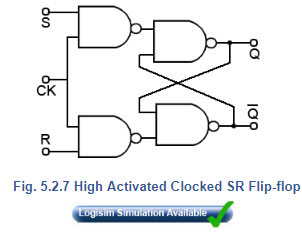## SR Flip-flop ICs

Comprising just two gates, low activated SR flip-flops are simple to implement using standard NAND gates but active low SR flip-flops (called SR flip-flops) are available as Quad packages in the LS TTL family as 74LS279 from Texas Instruments.

## Circuit Symbols for Flip-flops

Rather than drawing the schematic circuit for individual gate versions of flip-flops it is common to draw them in block form. Some commonly used block versions of SR and RS flip-flops are shown in Fig. 5.2.8.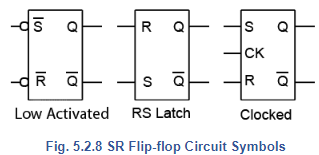The document RS Latch: S-R Flip Flops Notes | EduRev is a part of the Electrical Engineering (EE) Course Digital Electronics.
All you need of Electrical Engineering (EE) at this link: Electrical Engineering (EE)Use Code STAYHOME200 and get INR 200 additional OFF Use Coupon Code
All Tests, Videos & Notes of Electrical Engineering (EE): Electrical Engineering (EE)

### Top Courses for Electrical Engineering (EE)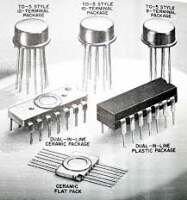## Digital Electronics

92 videos|91 docs|12 tests

### Top Courses for Electrical Engineering (EE)Track your progress, build streaks, highlight & save important lessons and more!

,

,

,

,

,

,

,

,

,

,

,

,

,

,

,

,

,

,

,

,

,

;